Processing ......FreeComputerBooks.com Links to Free Computer, Mathematics, Technical Books all over the World

Introduction to Computer Science
Related Book Categories:
•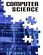Computer Science from the Bottom Up - From Operating Systems

It aims to teach computer science from the bottom end up - working from operating systems fundamentals through to how those applications are complied and executed, so you might be able to figure out where to start looking to make sense of it all.

•Computer Science Concepts in Scratch (Michal Armoni, et al)

This book will familiarize you with the Scratch visual programming environment. The book is structured as a collection of tasks. Concepts are introduced in order to solve a specific task such as animating dancing images or building a game.

•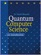Quantum Computer Science: An Introduction (David Mermin)

Quantum Physics has some spectacular applications in computer science. This book is a concise introduction to Quantum Computation, developing the basic elements of this new branch of computational theory without assuming any background in physics.

•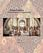Isomorphism - Mathematics of Programming (Xinyu Liu)

This book introduces the mathematics behind computer programming. It intents to tell: programming is isomorphic to mathematics. Just like in art and music, there are interesting stories and mathematicians behind the great minds.

•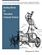Building Blocks for Theoretical Computer Science (Margaret Fleck)

For all readers interested in the basics of computer science. It provides a survey of basic mathematical objects, notation, and techniques which will be useful in later computer science courses.

•CS for All: An Introduction to Computer Science using Python

To provide an introduction to computer science as an intellectually rich and vibrant field rather than focusing exclusively on computer programming. It emphasizes concepts and problem-solving over syntax and programming language features.

•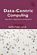A Data-Centric Introduction to Computing (Kathi Fisler, et al)

This book is an introduction to computer science. It will teach you to program, and do so in ways that are of practical value and importance. It uses a data-centric approach: data centric = data science + data structures.

•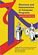Structure and Interpretation of Computer Programs using JavaScript

This book introduces the reader to central ideas of computation by establishing a series of mental models for computation. It has had a dramatic impact on computer science curricula over the past decades. Examples are implemented using the JavaScript.

•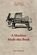A Machine Made this Book: Ten Sketches of Computer Science

This book is entertaining to read and gives a good basic introduction to the subject for anyone who hasn't studied Computer Science. It uses examples from the publishing industry to introduce the fascinating discipline of Computer Science to the uninitiated.

•Software Foundations (Benjamin C. Pierce, et al)

This book shows you how computer programming works without all the technical details or hard programming language. It explores the common parts of every computer programming language and how to write for multiple platforms.

•The Computer Science of TeX and LaTeX (Victor Eijkhout)

This book uses the TeX and LaTeX system to provide an introduction to a number of computer science topics. This book is based on the lecture notes of a course taught at the University of Tennessee.

•Logic for Computer Science: Automatic Theorem Proving

This book introduces mathematical logic with an emphasis on proof theory and procedures for algorithmic construction of formal proofs. It is useful for the formalization of proofs and basics of automatic theorem proving.

•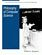Philosophy of Computer Science (William J. Rapaport)

This book concentrates on a tightly related group of topics which form the spine of the subject. These include specification, implementation, semantics, programs, programming, correctness, abstraction and computation.

•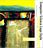Computer Science Logo Style (Brian Harvey)

This series is for people who are interested in computer programming because it's fun. It uses the Logo programming language as the vehicle for an exploration of computer science from the perspective of symbolic computation and artificial intelligence.

•Foundations of Computer Science, C Edition (Al Aho, et al)

This textbook combines the theoretical foundations of computing with essential discrete mathematics. It shows you how to use such essential data structures as lists, stacks, queues, sets, trees, heaps, priority queues, and graphs.

•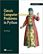Classic Computer Science Problems in Python (David Kopec)

This book deepens your knowledge of problem-solving techniques from the realm of computer science by challenging you with time-tested scenarios, exercises, and algorithms. As you work through examples in search, clustering, graphs, and more.

•Category Theory for the Sciences (David I. Spivak)

Using databases as an entry to category theory, this book explains category theory by examples, and shows that category theory can be useful outside of mathematics as a rigorous, flexible, and coherent modeling language throughout the sciences.

•Quantum Computing Since Democritus (Scott Aaronson)

This book takes readers on a tour through some of the deepest ideas of maths, computer science and physics. Full of insights, arguments and philosophical perspectives, the book covers an amazing array of topics.

•O'Reilly® Think Perl 6: How to Think Like a Computer Scientist

This practical guide gets you started on your programming journey with the help of Perl 6, the younger sister of the popular Perl programming language. Ideal for beginners, this hands-on book includes over 100 exercises with multiple solutions.

•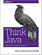O'Reilly® Think Java: How to Think Like a Computer Scientist

If you want to understand computer science, but have no programming experience, this book is for you. It starts with the most basic programming concepts and carefully defines every term when it's first used, in a logical progression.

•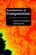Foundations of Computation (Carol Critchlow, et al)

It includes topics from discrete mathematics, automata theory, formal language theory, and the theory of computation, along with practical applications to computer science. The course has no prerequisites other than introductory computer programming.

•Computing Fundamentals: Theory and Practice of Software Design

The book introduces the reader to computer programming, i.e. algorithms and data structures. It covers many new programming concepts that have emerged in recent years including object-oriented programming and design patterns.

•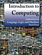Introduction to Computing: Language, Logic, and Machines

This book focuses on how to describe information processes by defining procedures, how to analyze the costs required to carry out a procedure, and the fundamental limits of what can and cannot be computed mechanically.

•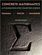Concrete Mathematics: A Foundation for Computer Science

This book introduces the mathematics that supports advanced computer programming and the analysis of algorithms. The primary aim of its well-known authors is to provide a solid and relevant base of mathematical skills.

•Discrete Mathematics for Computer Science (Jean Gallier)

This books gives an introduction to discrete mathematics for beginning undergraduates. One of original features of this book is that it begins with a presentation of the rules of logic as used in mathematics. Many examples of formal and informal proofs are given.

•Computer Science Tapestry: Exploring Programming in C++

This book is designed for a first course1 in computer science that uses C++ as the language by which programming is studied. It provides strong grounding in the analysis, construction, and design of programs and programming.

•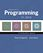Introduction to Computer Science (Robert Sedgewick, et al)

This book is for all readers interested in introductory programming courses using the Java programming language.

•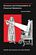Structure and Interpretation of Computer Programs, 2nd Edition

This book has had a dramatic impact on computer science curricula over the past decade. This long-awaited revision contains changes throughout the text. Examples are implemented using the Scheme dialect of Lisp.

•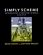Simply Scheme: Introducing Computer Science, 2nd Edition

This book works hard to make the somewhat tricky ideas of Scheme accessible. It stresses small sections on key language features, from basic functions, variables, and onward to recursion and other functional programming concepts.

•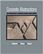Concrete Abstractions: An Intro to Computer Science Using Scheme

This book covers the basics of programming and data structures, and gives first-time computer science students the opportunity to not only write programs, but to prove theorems and analyze algorithms as well.

•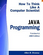How to Think Like a Computer Scientist: JAVA Programming

This book is an introduction to Java programming for beginners. It is tailored for students preparing for the Computer Science Advanced Placement (AP) Exam, but it is for anyone who wants to learn Java.

•How to Think Like a Computer Scientist, C++ Version (A. Downey)

The goal of this book is to teach you to think like a computer scientist, using C++ as the programming language. It means the ability to formulate problems, think creatively about solutions, and express a solution clearly and accurately.

•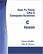How To Think Like A Computer Scientist: C Version

The topic of programming in the C language is vast, and the content selection represents a carefully selected minimal set of chapters which is both manageable for first-time programmers and a good basis for further growth.

•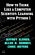How to Think Like a Computer Scientist: Learning with Python 3

This book is an introduction to computer science using the Python programming language. It covers the basics of computer programming, including variables and values, functions, conditionals and control flow, program development and debugging.

•Think Python - How to Think Like a Computer Scientist

Think Python is an introduction to Python programming for students with no programming experience. It starts with the most basic concepts of programming, and is carefully designed to define all terms when they are first used.

•Think OCaml: How to Think Like a Computer Scientist

This book is an introductory functional programming textbook based on the OCaml language. It is intended for newcomers who know some programming but want to learn programming in the function-oriented paradigm, or just want to learn OCaml.

Book Categories
 :All CategoriesTop Free BooksRecent BooksMiscellaneous BooksComputer EngineeringComputer LanguagesComputer ScienceData Science/DatabasesJava and Java EE (J2EE)Linux and UnixMathematicsMicrosoft and .NETMobile ComputingNetworking and CommunicationsSoftware EngineeringSpecial TopicsWeb Programming
Other Categories